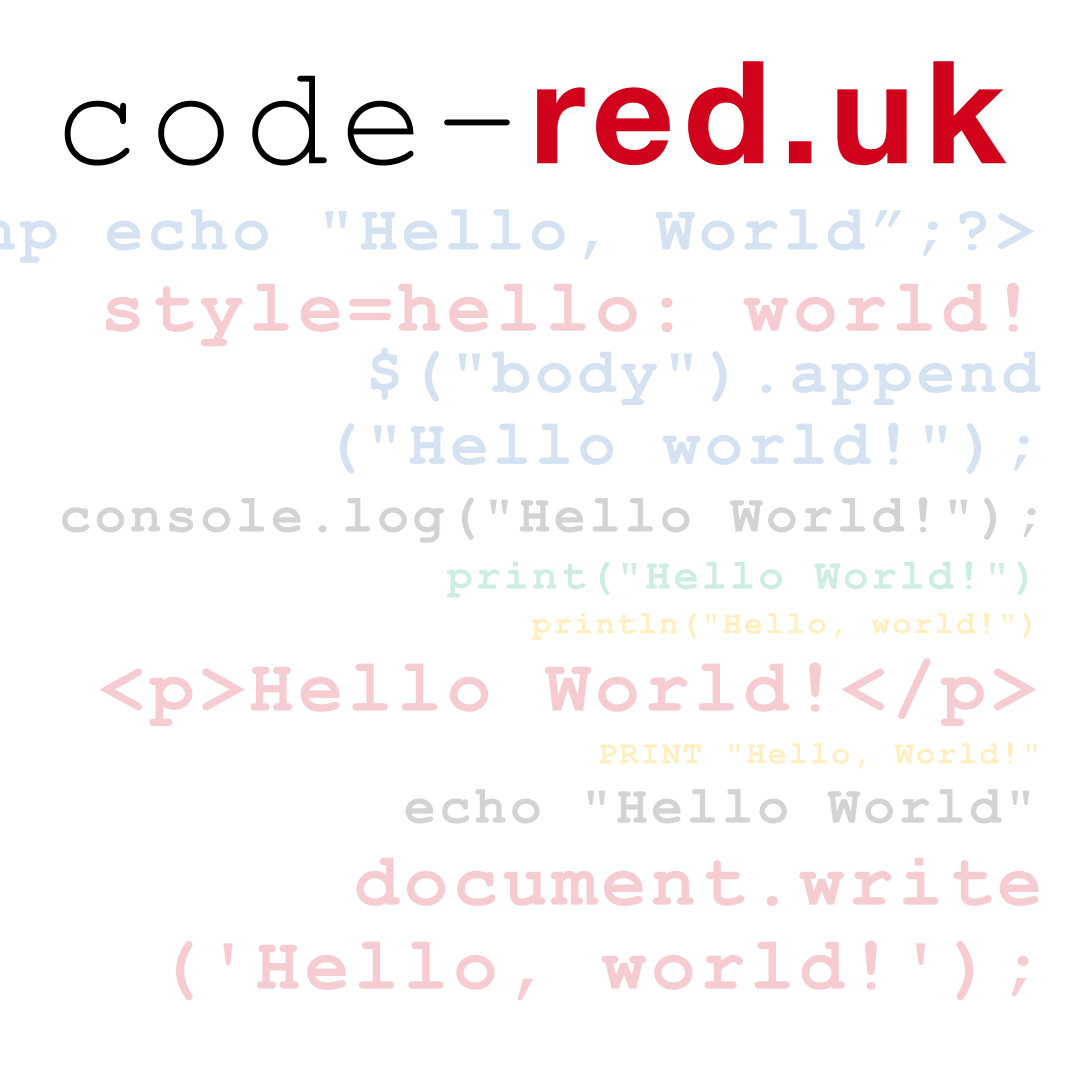So in JavaScript there a 3 types of equals `=` , `==` and `===` . So why so many and what are they for?

## `=` single equals

This 1 is easy to understand, it's used to set a variable. For example `var age = 50;` .

As we know there are 3 ways to declare a variable:

• `var`
• `let`
• `const`

The first 2, `var` & `let` , can be reassigned (changed), where as `const` can not and if you try to you'll get an error:

```Uncaught TypeError: Assignment to constant variable.
```

`var` is the old way of declaring variables, before the advent of ES6. Since then we have had 2 ways to declare variables `let` for variables that can change and `const` for variable that don't.

## `==` double equals & `===` triple equals

Both of these are for checking if a value is equal to something. For example is age equal to 50:

• `age == 50;`
• `age === 50;`

So why the need for both?

While it would appear that these two versions are the same they technically are not `===` triple equals is much stricter, so I'll start with that.

### `===` triple equals

As this is the stricter of the two types of equals, it checks not only the value but also the type of variable:

#### Variable as a number

```> let age = 50;
> age === 50;
< true
> typeof age;
< "number" ```

#### Variable as a string

```> let age = '50';
> age === 50;
< false
> typeof age;
< "string" ```

#### with `==` double equals

```> let age = '50';
> age == 50;
< true
> typeof age;
< "string" ```

It is recommended that `===` triple equals is used in the vast majority of cases as you'd want to make sure that not only the value is the same but also the type of variable is the same.

### `==` double equals

There is a use for the less strictness of == double equals and this is when we want to check if a varible does not have a value, either null or undefined:

```> let empty;
> empty;
< undefined;
> empty == undefined;
< true
> empty == null;
< true
```

This will return true for both `undefined` and `null` .

```> let empty;
> empty;
< undefined;
> empty === undefined;
< true
> empty === null;
< false```

Where as `===` triple equals will return false for null.

## Conclusion

• `=` single equals is used for setting the value of variables
• `==` double equals & `===` triple equals are used to check if a variable is set to something
• `===` triple equals use this, almost, always to check if a value equals something
• `==` double equals use this to check if a variable is either `undefined` OR `null`

## Other articles from the blog

•